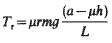Related Resources: mechanics machines

### Braking Torque Equation and Calculator

Mechanics And Machine Engineering and Design

Braking Torque Equation and Calculator:

Brake torque is the force applied at the brake wheel to stop the motion of the moving equipment.Assuming the operating conditions for the equipment are con- stant, a brake having a retarding torque equal to the full load torque of the motor to which it is applied is usually satisfactory.

Symbols used:
I = moment of inertia of a pair of wheels (kg-m2)
α = angular deceleration of wheels (m/sec2)
m = mass of vehicle (kg)
u = coefficient of friction between wheels and road (unitless)
g = gravity (m/sec2)
Tf = Torque (N-m)
Tbf = Torque Total Front (N-m)
Tbr = Torque Total Rear (N-m)

Preview: Braking Torque Calculator:

Braking Torque Basic Equation (Front wheels torque):Rear wheel Torque:Wheel Torque:Deceleration

d = ug

Total Braking Torque for one wheel.